Students can download Maths Chapter 3 Algebra Ex 3.11 Questions and Answers, Notes, Samacheer Kalvi 10th Maths Guide Pdf helps you to revise the complete Tamilnadu State Board New Syllabus, helps students complete homework assignments and to score high marks in board exams.

## Tamilnadu Samacheer Kalvi 10th Maths Solutions Chapter 3 Algebra Ex 3.11

Question 1.
Solve the following quadratic equations by completing the square method
(i) 9x2 – 12x + 4 = 0
9x2 – 12x + 4 = 0
x2 – $$\frac { 12x }{ 9 }$$ + $$\frac { 4 }{ 9 }$$ = 0 (Divided by 9)
x2 – $$\frac { 4x }{ 3 }$$ = $$\frac { -4 }{ 9 }$$
Add [$$\frac { 1 }{ 2 }$$ ($$\frac { 4 }{ 3 }$$)]2 on both sides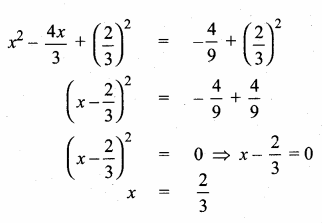The solution is $$\frac { 2 }{ 3 }$$(ii) $$\frac { 5x+7 }{ x-1 }$$ = 3x + 2
(3x + 2) (x – 1) = 5x + 7
3x2 – 3x + 2x – 2 = 5x + 7 ⇒ 3x2 – x – 5x – 2 – 7 = 0
3x2 – 6x – 9 = 0 ⇒ x2 – 2x – 3 = 0 (divided by 3)
x2 – 2x = 3
Adding ($$\frac { 1 }{ 2 }$$ × 2)2 on both sides
x2 – 2x + 1 = 3 + 1
(x – 1)2 = 4 ⇒ x – 1 = $$\sqrt { 4 }$$
x – 1 = ±2
x – 1 = 2 or x – 1 = -2
x = 3 or x = -1
The solution set is -1 and 3Question 2.
Solve the following quadratic equations by formula method
(i) 2x2 – 5x + 2 = 0
a = 2, b = -5, c = 2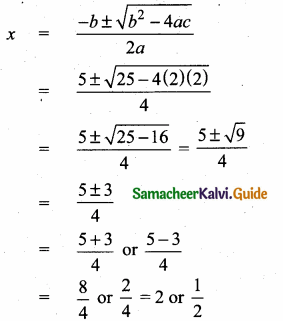The solution set is $$\frac { 1 }{ 2 }$$ and 2

(ii) $$\sqrt { 2 }$$ f2 – 6 f + 3 $$\sqrt { 2 }$$ = 0
Here a = $$\sqrt { 2 }$$, b = -6 and c = 3$$\sqrt { 2 }$$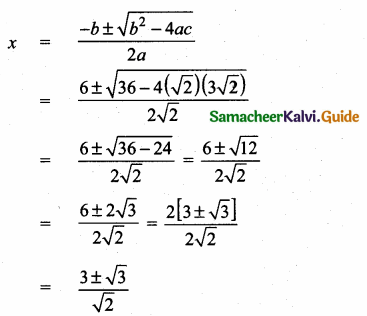The solution set is $$\frac{3+\sqrt{3}}{\sqrt{2}}$$ and $$\frac{3-\sqrt{3}}{\sqrt{2}}$$

(iii) 3y2 – 20y – 23 = 0
a = 3, b = -20, c = -23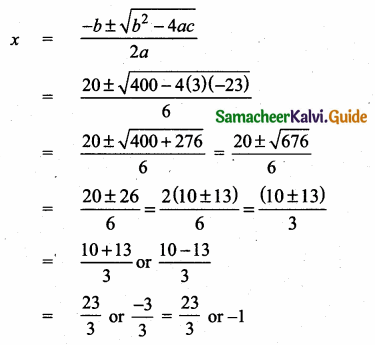The solution set is -1 and $$\frac { 23 }{ 3 }$$

(iv) 36y2 – 12ay + (a2 – b2) = 0
Here a = 36, b = -12a, c = a2 – b2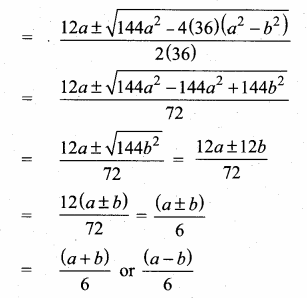The solution set is $$\frac { (a+b) }{ 6 }$$ and $$\frac { (a-b) }{ 6 }$$Question 3.
A ball rolls down a slope and travels a distance d = t2 – 0.75t feet in t seconds. Find the time when the distance travelled by the ball is 11.25 feet.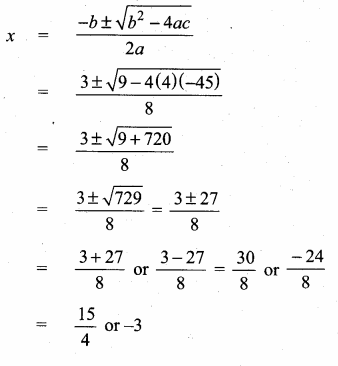The required time = $$\frac { 15 }{ 4 }$$ seconds
= 3 $$\frac { 3 }{ 4 }$$ second or 3.75 seconds